## Example Questions

### Example Question #121 : Algebra

Let f(x) = 2x3 + 7x2 - 4x and g(x) = 4x - x3. If k is a positive number such that f(k) = 0, then what is g(k)?

15/8

7/8

1

2

17/8

15/8

Explanation:

First, we need to find the possible values of k such that f(k) = 0.

We can use the definition of f(x) to write an expression for k.

f(x) = 2x3 + 7x2 – 4x

f(k) = 2k3 + 7k2 – 4k = 0

In order to solve this equation, we will want to factor as much as we can. We can immediately see that we could take out a k from all three terms.

2k3 + 7k2 – 4k = 0

k(2k+ 7k – 4) = 0

Now, we need to factor 2k+ 7k – 4 by grouping. When we multiply the outer two coefficients we get (2)(–4) = –8. The middle coefficient is 7. This means we need to think of two numbers that multiply to give us –8, but add to give us 7. These two numbers are 8 and –1. We can now rewrite 2k+ 7k – 4 as follows:

2k+ 7k – 4 = 2k2 + 8k – k – 4

Group the first two terms and the second two terms.

2k2 + 8k – k – 4 = (2k2 + 8k ) + (–k – 4)

Next, factor out a 2k from the first two terms and a –1 from the last two terms.

(2k2 + 8k ) + (–k – 4) = 2k(+ 4) + –1(+ 4)

Then, factor out + 4 from the 2k and the –1.

2k(+ 4) + –1(+ 4) = (+ 4)(2k – 1)

Thus, 2k+ 7k – 4, when fully factored, equals (+ 4)(2k – 1).

Now, let's go back to the equation k(2k+ 7k – 4) = 0 and substitute (+ 4)(2k – 1) for 2k+ 7k – 4.

k(2k+ 7k – 4) = k(+ 4)(2k – 1) = 0

We now have three factors, and we can set each equal to zero to find the possible values of k.

The first factor is k. This means k = 0 is one value for k.

The next factor is k + 4.

k + 4 = 0

k = –4

The last factor is 2k – 1.

2k – 1 = 0

2k = 1

k = 1/2

The values of k for which f(k) = 0 are 0, –4, and 1/2. However, we are told that k is positive, so this means that k can only be 1/2.

Ultimately, the problem asks us to find g(k). This means we must use the equation for g(x) to find g(1/2).

g(x) = 4x – x3

g(1/2) = 4(1/2) – (1/2)3

= 2 – (1/8)

= 15/8

### Example Question #121 : Algebra

The sum of two consecutive positive even integers is two greater than one-tenth of the product of the two integers. What is the sum of the positive factors of the smaller integer?

42

31

39

60

28

39

Explanation:

We need to translate the information given into an algebraic equation that will help us solve for the integers.

Let x represent the smaller of the two integers. Because they are consecutive even integers, the other integer is equal to x + 2.

We are told that the sum of the two integers is two greater than one-tenth of their product. We can represent the sum of the two integers as x + (+ 2).

To represent one-tenth of the product, we can write (1/10)(x)(+ 2). Two greater than this quantity would be equal to 2 + (1/10)(x)(+ 2). Remember that "two greater" requires us to add 2, while "two times greater" would have required us to multiply by 2.

Now, we can set the sum equal to two greater than one-tenth the product.

x + (+ 2) = 2 + (1/10)(x)(+ 2)

Multiply both sides by 10 to eliminate the fraction.

10(x + x + 2) = 10(2 + (1/10)x(+ 2))

Distribute the 10 on both sides.

10x + 10x + 20 = 20 + 10(1/10)x(+ 2)

Simplify both sides.

20x + 20 = 20 + x(+ 2)

Distribute the x on the right side.

20x + 20 = 20 + x+ 2x

Subtract 20 from both sides.

20x = x2 + 2x

Subtract 20x from both sides.

x– 18x = 0

Factor out an x.

x(x – 18) = 0

Set each factor equal to zero.

x = 0, or x – 18 = 0, which means x = 0 or 18. However, because we are told that the integers are positive, the value of x must be 18.

The smaller integer is 18. We are asked to find the sum of its factors. To find the factors of 18, we must think of all the pairs of numbers that will multiply to give us 18. The pairs are as follows:

1 and 18, 2 and 9, 3 and 6

This means the factors of 18 are 1, 2, 3, 6, 9, and 18. The sum of these numbers is 1 + 2 + 3 + 6 + 9 + 18 = 39.

### Example Question #1801 : Sat Mathematics

In a group of 24 children, there are twice as many girls as there are boys.  How many boys are there?

8

12

10

16

14

8

Explanation:

Since there are twice as many girls as boys, we know that 2b = g.

Since there are 24 total, we know that b + g = 24.

Substituting the first equation into the second equation yields

b + 2b = 24

3b = 24

b = 8

### Example Question #124 : Algebra

For each megawatt of power that it produces, a power plant spends $30,000 on coal,$50,000 on salaries, and $20,000 on general expenses. If the plant produces 250 megawatts, how much does it spend on salaries and general expenses combined? Possible Answers:$15,000,000

$20,000,000$12,500,000

$17,500,000 Correct answer:$17,500,000

Explanation:

The problem is simple if you do not mix up your values and their meanings. What we know is that for 250 megawatts, we should have 250 times the expenses in each of our categories. Therefore, for our data, we know the following.

For salaries, we have $50,000 * 250 or$12,500,000.

For general expenses, we have $20,000 * 250 or$5,000,000

Therefore, our total is: $12,500,000 +$5,000,000 = $17,500,000 We also could have calculated this by adding$50,000 and $20,000 together to get$70,000 per megawatt. This would be $70,000 * 250 =$17,500,000 as well.

### Example Question #125 : Algebra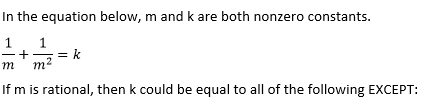24

12

6

20

2

24

Explanation: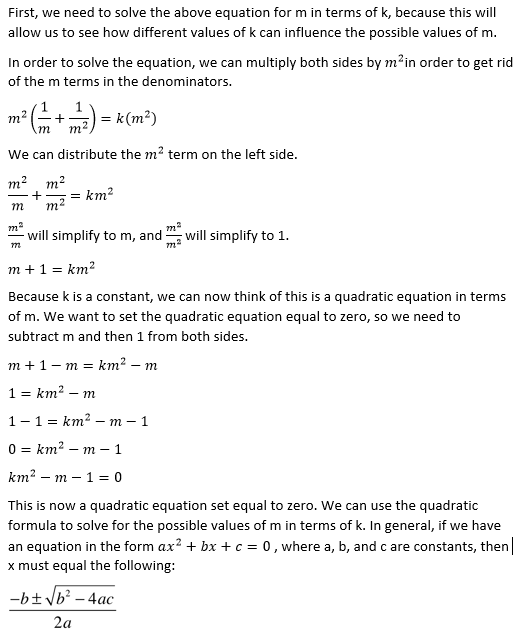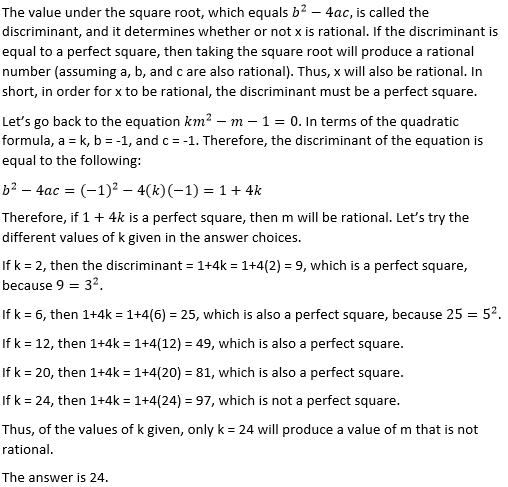### Example Question #121 : Algebra

For what value of x does 4(3x – 2) = 12?

5/6

1

5/3

2

2/3

5/3

Explanation:

We have to solve the equation 4(3x – 2) = 12. First, we can distribute the left side.

4(3x) – 4(2) = 12

12x – 8 = 12

Then we add 8 to both sides.

12x = 20

Divide both sides by 12.

x = 20/12

Simplify 20/12 by dividing the numerator and denominator by 4.

x = 20/12 = 5/3

### Example Question #21 : Equations / Inequalities

If 11 + 3x is 29, what is 2x?

2

36

12

6

12

Explanation:

First, solve for x:

11 + 3= 29

29 – 11 = 3x

18 = 3x

x = 6

Then, solve for 2x:

2= 2 * 6 = 12

### Example Question #82 : Linear / Rational / Variable Equations

If 2x = 3y = 6z = 48, what is the value of x * y * z?

6144

1024

2304

1536

3072

3072

Explanation:

Create 3 separate equations to solve for each variable separately.

1) 2x = 48

2) 3y = 48

3) 6z = 48

x = 24

y = 16

z = 8

* y * z = 3072

### Example Question #61 : How To Find The Solution To An Equation

If 3|x – 2| = 12 and |y + 4| = 8, then |x - y| can equal ALL of the following EXCEPT:

14

10

2

18

6

14

Explanation:

We must solve each absolute value equation separately for x and y. Remember that absolute values will always give two different values. In order to find these two values, we must set our equation to equal both a positive and negative value.

In order to solve for x in  3|x – 2| = 12,

we must first divide both sides of our equation by 3 to get |x – 2| = 4.

Now that we no longer have a coefficient in front of our absolute value, we must then form two separate equations, one equaling a positive value and the other equaling a negative value.

We will now get x – 2 = 4

and

x – 2 = –4.

When we solve for x, we get two values for x:

x = 6 and x = –2.

Do the same thing to solve for y in the equation |y + 4| = 8

and we get

y = 4 and y = –12.

This problem asks us to solve for all the possible solutions of |x - y|.

Because we have two values for x and two values for y, that means that we will have 4 possible, correct answers.

|6 – 4| = 2

|–2 – 4| = 6

|6 – (–12)| = 18

|–2 – (–12)| = 10

### Example Question #1 : How To Find Out When An Equation Has No Solution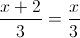Solve for.

No solutions.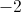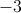Cross multiplying leaves, which is not possible.